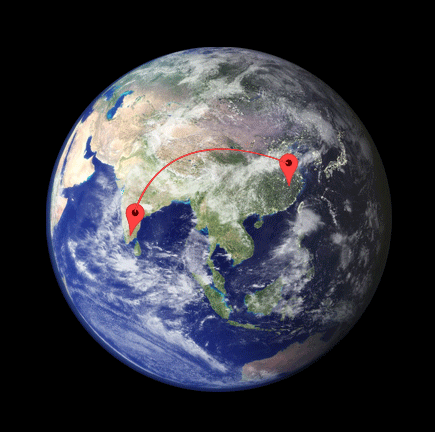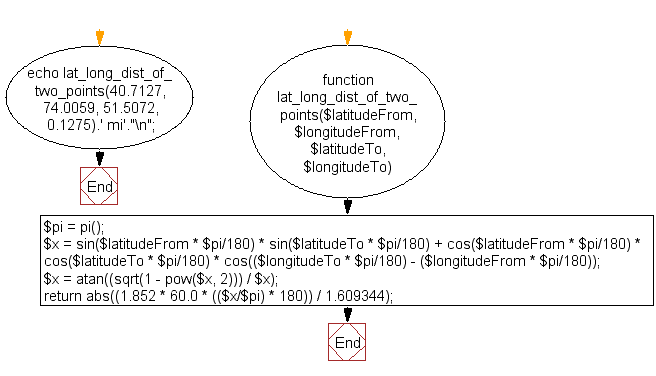﻿ PHP Math Exercise: Get the distance between two points on the earth - w3resource# PHP Math Exercises : Get the distance between two points on the earth

## PHP math: Exercise-12 with Solution

Write a PHP function to get the distance between two points on the earth.

Pictorial Presentation:Sample Solution:

PHP Code:

``````<?php
function lat_long_dist_of_two_points(\$latitudeFrom, \$longitudeFrom, \$latitudeTo, \$longitudeTo){
\$pi = pi();
\$x = sin(\$latitudeFrom * \$pi/180) *
sin(\$latitudeTo * \$pi/180) +
cos(\$latitudeFrom * \$pi/180) *
cos(\$latitudeTo * \$pi/180) *
cos((\$longitudeTo * \$pi/180) - (\$longitudeFrom * \$pi/180));
\$x = atan((sqrt(1 - pow(\$x, 2))) / \$x);
return abs((1.852 * 60.0 * ((\$x/\$pi) * 180)) / 1.609344);
}
// Distance from New York to London
echo lat_long_dist_of_two_points(40.7127, 74.0059, 51.5072, 0.1275).' mi'."\n";
?>
```
```

Sample Output:

```3458.8601748984 mi
```

Flowchart :PHP Code Editor:

Have another way to solve this solution? Contribute your code (and comments) through Disqus.

What is the difficulty level of this exercise?

﻿

## PHP: Tips of the Day

SQL injection that gets around mysql_real_escape_string()

Consider the following query:

```\$iId = mysql_real_escape_string("1 OR 1=1");
\$sSql = "SELECT * FROM table WHERE id = \$iId";
```

mysql_real_escape_string() will not protect you against this. The fact that you use single quotes (' ') around your variables inside your query is what protects you against this. The following is also an option:

```\$iId = (int)"1 OR 1=1";
\$sSql = "SELECT * FROM table WHERE id = \$iId";
```

Ref : https://bit.ly/32q3bJ7